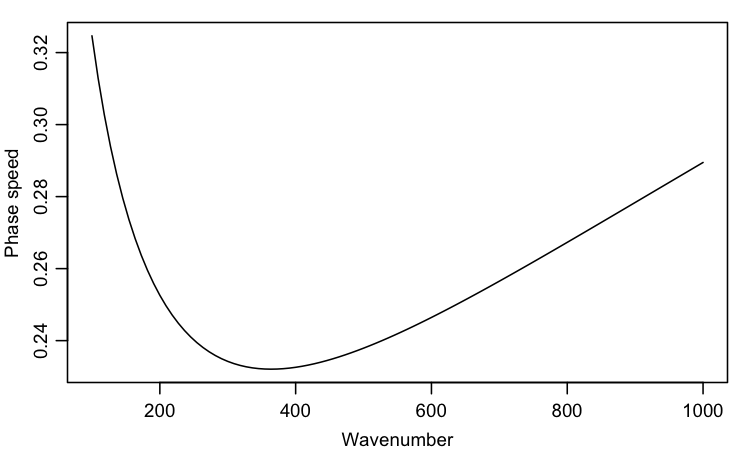# Introduction

R provides functions for both one-dimensional and multi-dimensional optimization. The second topic is much more complicated than the former (see e.g. Nocedal 1999) and will be left for another day.

A convenient function for 1D optimization is optimize(), also known as optimise(). Its first argument is a function whose minimum (or maximum) is sought, and the second is a two-element vector giving the range of values of the independent variable to be searched. (See ?optimize for more.)

# Application

As an example, consider the phase speed of deep gravity-capillary waves, which is given by $\omega/k$ where $\omega$ is the frequency and $k$ is the wavenumber, and the two are bound together with the dispersion relationship $\omega^2=gk+\sigma k^3/\rho$, where $g$ is the acceleration due to gravity, $\sigma$ is the surface tension parameter (0.074 N/m for an air-water interface) and $\rho$ is the water density (1000 kg/m^3 for fresh water). This yields wave speed given by the following R function.

It makes sense to plot a function to be optimized, if only to check that it has been coded correctly, so that is the next step. Readers who are familiar with gravity-capillary waves may know that the speed is minimum at wavelengths of about 2 cm, or wavenumbers of approximately $2\pi/0.02=300$; this suggests an x range for the plot.The results suggest that the range of $k$ illustrate contains the minimum, so we provide that to optimize().

This speed is not especially fast; it would take about a heartbeat to move past your hand.

# Exercises

1. Use str(o) to learn about the contents of the optimized solution.

2. Use abline() to indicate the wavenumber at the speed minimum.

3. Try other functions that are of interest to you, e.g. find the angle that maximizes $\sin\theta\cos\theta$, which yields the throwing angle that achieves furthest distance in frictionless air over flat terrain.

4. Use the multi-dimensional optimizer named optim() on this problem.

Jorge Nocedal and Stephen J. Wright, 1999. Numerical optimization. Springer series in operations research. Springer-Verlag, New York, NY, USA.